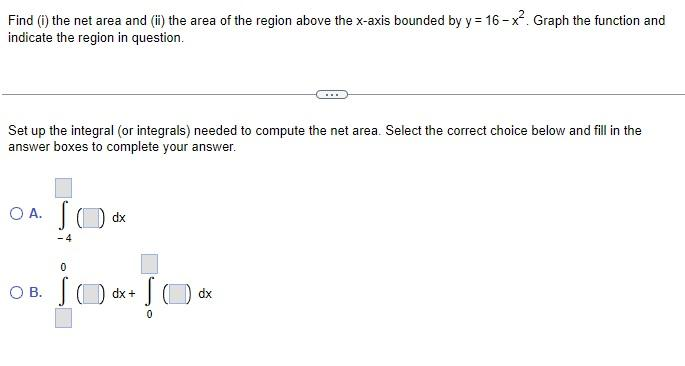Home / Expert Answers / Calculus / find-i-the-net-area-and-ii-the-area-of-the-region-above-the-x-axis-bounded-by-y-16-x-pa289

# (Solved): Find (i) the net area and (ii) the area of the region above the $$x$$-axis bounded by $$y=16-x^ ...Find (i) the net area and (ii) the area of the region above the \( x$$-axis bounded by $$y=16-x^{2}$$. Graph the function and indicate the region in question. Set up the integral (or integrals) needed to compute the net area. Select the correct choice below and fill in the answer boxes to complete your answer. A. $$\int_{-4}(\square) d x$$ B. $$\int_{0}^{0} 1 \mid d x+\int_{0}(1 d x$$

We have an Answer from Expert